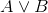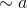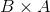Varsity Tutors always has a different Symbolic Logic Question of the Day ready at your disposal! If you’re just looking to get a quick review into your busy day, our Symbolic Logic Question of the Day is the perfect option. Answer enough of our Symbolic Logic Question of the Day problems and you’ll be ready to ace the next test. Check out what today’s Symbolic Logic Question of the Day is below.

### Question of the Day: Symbolic Logic

Which of the following statements is part of the definition for sentential logic?

Every lower case letter is a formula.

Ifandare formulas thenis a formula as well.

Ifis a formula then so is.

Anything and everything can be considered a formula.

Ifis a formula then so is.

### All Symbolic Logic Resources